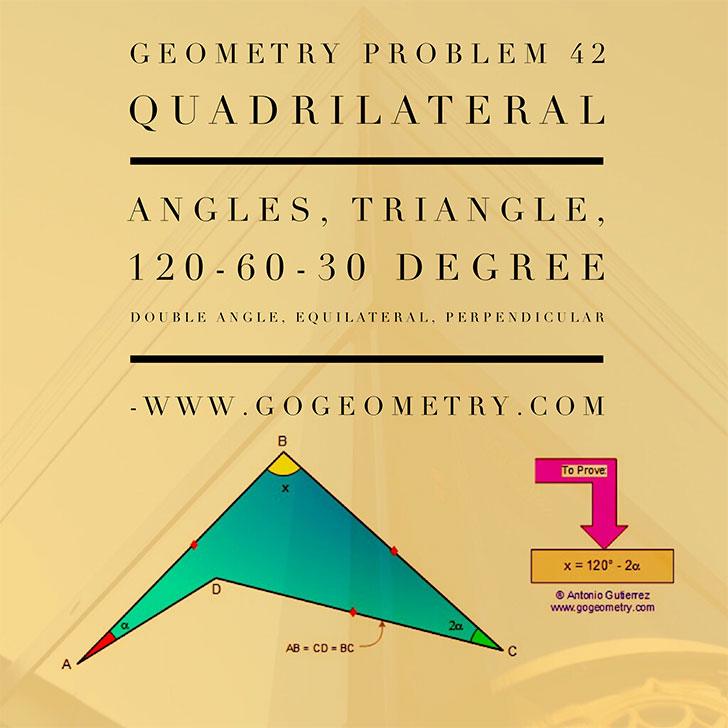# Geometry Problem 42 with Solution: Angles, Triangle, Isosceles, Quadrilateral, Congruence, Double Angle, 120 Degrees

< PREVIOUS PROBLEM  |  NEXT PROBLEM >

In the figure, AB = BC = CD, and the measure of angle C is double the measure of angle A. Prove that x = 120° -2mÐ A.Home | Geometry | Problems | Open Problems | 10 Problems | 41-50 | Double Angle | Email | by Antonio Gutierrez Post or view a solution to the problem 42